Biomedical Sciences
Volume 2, Issue 5, September 2016, Pages: 34-47

Review Article

Towards Physical Understanding of Molecular Recognition in the Cell: Recent Evolution of Molecular Dynamics Techniques and Free Energy Theories

Takefumi Yamashita

Laboratory for Systems Biology and Medicine, Research Center for Advanced Science and Technology, the University of Tokyo, Tokyo, Japan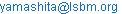Takefumi Yamashita. Towards Physical Understanding of Molecular Recognition in the Cell: Recent Evolution of Molecular Dynamics Techniques and Free Energy Theories. Biomedical Sciences. Vol. 2, No. 5, 2016, pp. 34-47. doi: 10.11648/j.bs.20160205.11

Received: September 29, 2016; Accepted: October 19, 2016; Published: November 21, 2016

Abstract: In cells, molecules do not arbitrarily interact with others; interact only with molecules of a particular type. This molecular recognition is a very important molecular function as one of the molecular bases on which the cells sustain their lives. Recently, it has been found that molecular recognition, which occurs not only between protein and protein but also between RNA and protein, plays important roles in the cell. Understanding of the molecular recognition at the atomic level is one of the challenging problems in the field of molecular biology and biochemistry. In this review, we address the theoretical and practical aspects of molecular dynamics simulation, which has become an important tool for studying the molecular recognition. From the theoretical viewpoint, many free energy calculation methods based on statistical mechanics have been developed. As for the practical aspects, it is important that the evolution of the computing technique not only enabled long-time simulations, but also enhanced prediction accuracy of simulations with developing new reliable force fields. By the recent development of theory and technology, the challenging tasks such as analysis and prediction of conformational distribution, structural change, and free energy of protein and/or nucleic acid systems are becoming possible.

Keywords: Molecular Dynamics, Binding Free Energy, Simulation, Molecular Recognition, Protein, RNA

1. Introduction

Many types of molecules are present in a cell and the function of the cells is expressed by their interaction. However, molecules do not arbitrarily interact with others but only with molecules of a particular type . Due to this molecular recognition, the cells can perform the orderly biological activity with maintaining the interaction network. Thus, this molecular recognition is a very important molecular function as one of the molecular bases on which the cells express their functions. Recently, it has been found that molecular recognition, which occurs not only between protein and protein but also between RNA and protein, plays an important role in the cell .

Understanding of molecular recognition at the atomic level is one of the challenging problems in the field of molecular biology and biochemistry. To quantify the molecular recognition, the binding free energy is the most important physical quantity. In addition to the interaction energy calculated from given structures, the binding free energy is also affected by the entropy involving dynamic effects. Therefore, in order to understand the molecular recognition at the atomic level, it is important to describe the dynamics of wandering through various conformations as well as to precisely describe a particular conformation of biomolecules. As an important tool for such analyses, molecular dynamics (MD) simulation is expected to be a promising one. This review addresses the theoretical and practical aspects of MD simulation related to analysis of molecular recognition. From the theoretical point of view, many free energy calculation methods based on the theory of statistical mechanics have been developed. As for the technical aspects, the evolution of the computing technique not only enabled long-time simulations, but also higher accuracy of the force field, which inherently improves the prediction of the physical quantity. It is essential to reproduce and analyze the long-time scale structural change dynamics of protein associated with the molecular recognition.

The evolution of MD simulation technology is also expected to accelerate the drug development process in the near future . Currently, a number of software programs developed for drug design include the function of approximate calculation of the binding free energy, according to which drug candidates are selected from many compounds. These approximate calculation methods utilize only one structure of the protein (in most cases, a crystal structure), while the effect of dynamic structural changes of a protein in water (in particular, those related to entropy) is not taken into account. Furthermore, water molecules are not explicitly considered, either. With these approximations, the free energy calculation becomes simple and fast enough to analyze many compounds even with a personal computer. However, such binding free energy predictions often have large errors. Therefore, as a new technology for the next generation, we expect to apply the all-atom MD simulation technique to the drug design. In the all-atom MD simulation, not only the target proteins and/or compounds, but also water molecules and salts are described at the atomic level and the behavior of the system is naturally reproduced on the computer. This point is a crucial difference from the free energy evaluation methods involved in the conventional drug design software.

The use pattern of MD calculations can be roughly categorized into two. Firstly the MD simulation is used as a "high-resolution microscope." The MD simulation can visualize the behavior of atoms, which cannot be observed directly in the experiments. Recently, Shaw et al.  developed a special-purpose computer "Anton" capable of nearly millisecond-scale MD simulation, and incidentally the computer is named after Antonie van Leeuwenhoek, who paved the way for microbial research by improving the microscope. The second type of usage is that to obtain information of a virtual system that does not exist in reality. The value of the information about a system non-existent in reality is mainly a more efficient calculation of physical quantities. To estimate the binding free energy by the normal MD simulation, interminably long simulation is required to sample events of binding and dissociation between the molecule and the protein. For example, since the dissociation rate constant of HIV protease and the inhibitor saquinavir is in the order of 10-4 s-1 , the dissociation process cannot be reproduced by MD simulation even with the latest supercomputer. However, by taking advantage of the information of a virtual system (in the case of MP-CAFEE calculations performed in our studies), the binding free energy can be accurately obtained from the information of ca. 1 µs in total.

In the next section, several important theories fundamental to the second usage are explained and addressed. There is a long history of theoretical constructions for coupling virtual system calculations and free energy, and new theories for improvement still continue to be proposed. The topic is not simply to establish statistical mechanics theories by making connection between motions of virtual systems and free energy via mathematical formulae, but statistical sampling efficiency is also important to predict how accurate physical quantities can be obtained from a limited calculation. In the third section, we discuss the first usage to understand the structural change dynamics of biomolecules, which is often essential to the molecular recognition. Currently, by increasing the accuracy of the MD simulation via the development of novel force fields as well as the advancement of computing power, it has become possible to reproduce slow structural changes of biological molecules. Based on several examples, we will discuss what insight can be extracted from the MD simulation. Finally, we provide concluding remarks in Section 4.

2. Theories of Free Energy Calculation

2.1. Alchemical Free Energy Calculation Method

The term "alchemical free energy calculation method" [6,7] often appears in many scientific articles in these years. This is a collective term of the thermodynamic integration (TI) method, the free energy perturbation (FEP) method, etc., and the word "alchemical" implies the use of an imaginary state as discussed above.

As an example, let us consider the process of annihilating the interaction of a ligand in aqueous solution. By dividing the Hamiltonian H of the aqueous system dissolving one ligand into the term V expressing the interaction of the ligand with other molecules and the other term H0, a scaling parameter λ is introduced into the former.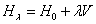(1)

Obviously, when λ = 1, Hλ becomes the Hamiltonian H of the real system. When, on the other hand, λ = 0, the ligand disappears from the original system, corresponding to a state without any interaction, i.e., to a state of an isolated ligand, such as in the gas phase. Thus, the change of λ from 1 to 0 corresponds to the state change: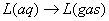(2)

Here, L denotes the ligand, and aq and gas mean aqueous solution state and gas phase state, respectively. The change in free energy associated with the reverse state change is called solvation free energy.

A computer simulation enables the free energy calculation by changing the parameter λ adequately. In reality, however, there is no intermediate state corresponding to λ = 0.5. Thus, one of the great advantages of the computer science is that it can investigate systems that do not exist in reality to derive a physical property of the real system.

To calculate the binding free energy between a ligand and a protein, similarly to the above example, the scaling parameter λ is introduced into the interaction term relative to the ligand in the total Hamiltonian representing the ligand-protein complex system in aqueous solution. When λ = 1, Hλ represents the ligand-protein complex system in aqueous solution. On the other hand, when λ = 0, Hλ corresponds to the state where only protein remains in the aqueous solution and, separately, the ligand is in the state of a gas phase. Therefore, a change of λ from 1 to 0 corresponds to the state change of the system: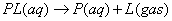(3)

Subtracting Eq. (2) from Eq. (3),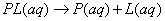(4)

is obtained. This equation represents the transition from the binding state to the dissociated state in solution. The corresponding free energy change is referred to as binding free energy. Thus, the binding free energy can be calculated as the difference between the free energy change of Eq. (3) and the free energy change of Eq. (2). In this binding free energy calculation, the free energy calculation corresponding to annihilation of the ligand is carried out twice, and therefore this method is called double annihilation .

In the following section, detailed explanation is given about how to calculate the free energy change due to state changes according to Eqs. (2) or (3).

2.2. Thermodynamic Integration Method

First of all, we explained the TI method . Here, in an equation similar to Hamiltonian of Eq. (1)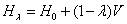(5)

the transition of λ from state 0 to state 1 is assumed. Although Eq. (5) is essentially the same as Eq. (1), but the difference from Eq. (1) is that interaction is fully included when λ = 0 and disappears when λ = 1. Here, the free energy difference can be written as: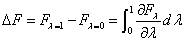(6)

On the other hand, the free energy and Hamiltonian can be linked by the relational expression: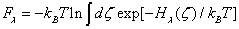(7)

Here, kB is the Boltzmann constant, T is the temperature, and ζ=(q,p) are phase space coordinates (positions and momenta of all particles). In combination with Eq. (6), the following equation is obtained: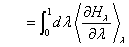(8)

This is the fundamental equation of the TI method. Here, <..> λ denotes the ensemble average in the equilibrium state of the system governed by the Hamiltonian Hλ.

As a simple idea to actually calculate Eq. (8), considering λ as a function of time, MD simulation can be carried out, through the following equation: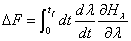(9)

while changing the λ from 0 to 1 monotonically. This is so called slow growth method. However, in order to make the calculation of formula (8) with Eq. (9), it is required to maintain equilibrium at each time and sufficient sampling must be performed before λ substantially changes. In particular, the case when the former condition is not satisfied is referred to as Hamiltonian lag problem .

There is also the method of integrating Eq. (8) numerically by discretizing the parameter λ. Here, for simplicity, equidistant discretization is performed. Thus,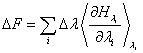can be obtained. To obtain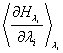,

one just need to perform MD calculation (or Monte Carlo calculation) of the system with a Hamiltonian Hλi and to averageover the sampled coordinates. It may be a difficult issue to sufficiently equilibrate the system and sufficiently converge the ensemble average in each λi.

2.3. Free Energy Perturbation Method

Formally, the free energy difference of two states can be described more directly. The basic equation for FEP method has been derived by Zwanzig  in 1954. In the Hamiltonian system governed by Eq. (1), the free energy of the system with λ = 1 can be expressed as follows: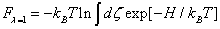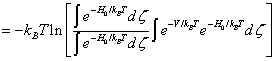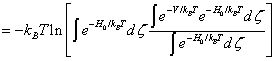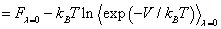(10)

Thus, the free energy difference can be written as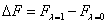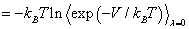(11)

This is the basic equation of the FEP method. In general, the free energy difference from system A to system B can be calculated by the ensemble average in the system A: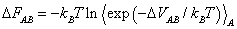(12)

Here, ΔVAB is the difference between the potential energy of the system A and system B in the same particle arrangement. Note that no approximation is contained at the stage of Eq. (12).

While in Eq. (8), the basic equation of the TI method, the free energy difference is calculated as an integral with respect to continuous change of the parameter λ, it is directly calculated only from the sampling in system A in Eq. (12).

However, such a primitive FEP calculation rarely provides an accurate prediction of free energy difference. One of the reasons for this is the ensemble average of the exponential function in Eq. (12). In principle, an accurate free energy value should be obtained if the structures were sampled infinitely; in reality, the calculation must be performed with finite samples. This is an interesting and fascinating point of molecular simulation not encountered in the pure theoretical science. If the distribution of ΔVAB is broad, only the low-energy side tail determines the free energy. In other words, most of the ΔVAB to be sampled in the molecular simulation do not play a dominant role in the free energy calculation. Therefore, the majority of ΔVAB important in the calculation of the free energy is not, or cannot be, sampled in the real MD simulation. To accurately calculate the free energy by the FEP method, in order to diminish the distribution of ΔVAB, it is necessary to calculate by dividing the λ into small steps.

For a high temperature state (|ΔVAB/kBT| << 1), Zwanzig also derived an approximate equation based on the second order perturbation expansion,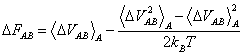(13)

Although the average of exponential function disappears, it is still necessary to discretize the change of λ into small steps for the accurate free energy calculation because of the strong constraint of |ΔVAB/kBT| << 1. Although Eq. (12) is exact, one may need to add more ingenuity to the calculation process for accurate free energy calculation with finite samples.

2.4. Jarzynski Equality

In 1997, Jarzynski has proposed an equation (Jarzynski equality)  which connects works of nonequilibrium processes to the free energy difference. It is highly interesting that this equation allows us to understand the TI and FEP methods systematically through the concept of "work". (Note that the following proof of Jarzynski equality is basically the same as in Ref. .)

Jarzynski equality assumes a situation where a system of interest is coupled with a heat bath while the entire system is isolated. Here, the system of interest represented by Eq. (1) is in contact with a heat bath having a Hamiltonian HB (η). η is the phase space coordinates of the heat bath. The total system (= system + heat bath) is isolated and the Hamiltonian can be given by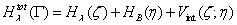(14)

Here, Vint represents the interaction between the system and the heat bath, Γ = (ζ, η). The whole system is assumed to reach thermal equilibrium in the initial state. Since the partition function of the total system is(15)

the appearance probability of a phase space point Γ in the initial state (λ = 0) is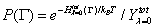(16)

For this initial condition, increasing the parameter λ monotonically as a function of time, the work is done on the system. (Here, λ = 0 at t = 0 and λ = 1 at t = τ.) The change in internal energy of the system for this work is represented as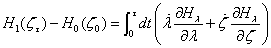(17)

Here, ζt is the coordinate ζ of the system at time t and represents a single path for the time evolution in accordance with equation of motion of the system starting from ζ0. Assuming the work done on the system as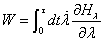(18)

the heat absorbed by the system is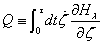(19)

These equations define W and Q only in dynamical operation of a partial system, but Eq. (17) can be still regarded as the first law of thermodynamics. The change in internal energy of the total system is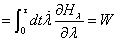(20)

which is equal to the work done on the system of interest. Since the partial differential in Γ becomes zero according to Liouville theorem for an isolated system and the λ-dependence of the Hamiltonian of the total system is solely the λ-dependence of the Hamiltonian of the system of interest, Eq. (20) can be derived.

Since in the initial state the total system has a distribution of the thermal equilibrium state, the average of the exponential function of the work is: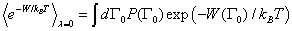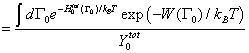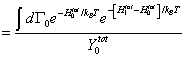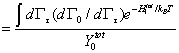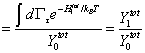(21)

indicating the ratio of the partition function in the initial state to that in the final state of the total system. Importantly, Eq. (21) is derived independently of whether the system of interest or the total system remains in equilibrium at time τ. Also, this equation is independent of the time evolution of the parameter λ. In Eq. (21), terms from lines 1 to 2 are obtained by only substituting the definition of formula (16). W0) explicitly expresses that the work depends on the initial conditions. From lines 2 to 3, Eq. (20) is used. In line 4, the Γ0 integral is replaced with Γτ integral with using the fact that the Jacobian dΓ0/dΓτ is 1 in accordance with the Liouville theorem for an isolated system.

On the other hand, in an equilibrium state of the total system, the probability that the system is found in ζ is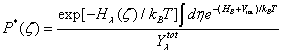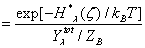(22)

Here, note that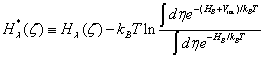(23)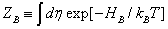(24)

Here, the denominator of the second term of Eq. (23) is introduced so as to obtain Hλ*=Hλ when Vint=0. The partition function corresponding to Eq. (22), assuming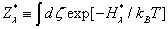(25)

can be written as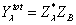(26)

Because ZB is independent of λ, Eq. (21) can be rewritten as: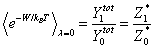(27)

Furthermore, since in the limit of Vint=0, Eq. (25) takes the form of(28)

the free energy difference of the system of interest can be represented as(29)

Consequently, Eq. (27) is obtained: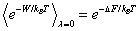(30)

This Eq. (30) is the Jarzynski equality. It is important that any approximation is excluded in this derivation and Eq. (30) is an exactly established theoretical formula. Even if work is carried out in a nonequilibrium process, it is related to the free energy difference between two equilibrium states.

In the case λ varies in a quasi-static process, Eq. (30) becomes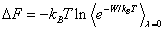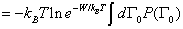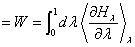(31)

Once trying to write explicitly the ensemble average as in the second line, Γ0-dependence on W disappears in a quasi-static process and can be written as in line 3. Then, it is straightforward to obtain line 4, which is the same as Eq. (8), the basic formula of the TI method, indicating that the Jarzynski equality (30) encompasses the TI method.

In the case of adiabatic change with infinitely rapid change of λ, the process is completed instantaneously, and the work is simply equal to the difference of Hamiltonian difference (W = V = ΔH = H1-H0). Thus, Eq. (30) can be rewritten as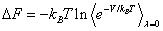(32)

This is identical with the basic equation of the FEP method [Eq. (11)]. While the proof of Zwanzig only associates the free energy difference between the two states with the difference of the Hamiltonian mathematically, the proof using the Jarzynski equality associates the free energy difference with the physical processes under the adiabatic and non-equilibrium condition.

The Jarzynski equality provides a comprehensive understanding of the TI method and FEP method, although the basic equation of the TI method looks just different from that of the FEP method. The two methods correspond to the two opposite limits of physical processes; the TI method implies quasi-static change, whereas an instantaneous adiabatic change is considered in the FEP method. Although both the methods are correct from a pure theoretical viewpoint, it is important to consider which one is capable to efficiently provide the accurate free energy prediction with limited computer resources from the viewpoint of computational science. In this context, one key would be to consider whether the molecular simulation reproduces the physical process underlying the free energy calculation. It may be more difficult to simulate a quasi-static process accurately in computer than to reproduce an instantaneous adiabatic transition. Therefore, it seems easier to properly perform the FEP calculation than the primitive TI calculation through MD simulation. In the case of the slow growth method, the problem of Hamiltonian lag  occurs frequently. This indicates that the equilibration of the system cannot follow the changes of λ in the simulation and the quasi-static process assumed in the theory is not completely realized. Therefore, it should be difficult to obtain the accurate free energy prediction by using the MD simulation inducing the Hamiltonian lag.

2.5. Bennett Acceptance Ratio Method

The Jarzynski equality derives the free energy difference from the work associated with the transition from state 0 to state 1. However, the reverse transition from the state 1 to 0 also exists in principle. The Bennett acceptance ratio (BAR) method  takes advantage of the information of transitions in both directions aiming at improving the accuracy. (Not written explicitly in the original paper of Bennett, but this would be the spirit of the BAR method. In the derivation of Shirts et al., to be introduced later , this can be seen more clearly.)

In Bennett’s paper, the basic equation is derived by minimizing the error of the free energy difference to be calculated. First, the free energy change associated with the transition from state 0 to state 1 can be written as: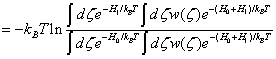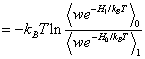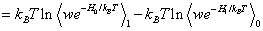(33)

As shown by line 3 and 4, sampling is assumed both in state 0 and state 1. Here, w(ζ) is a weighting function, which will be determined later to minimize the square of the difference between the statistically estimated value ΔFest and the true value ΔF (variance).

The true average of wexp(-H0/kBT) for the ensemble of λ = 1 is denoted by m1, and then the first term of the last line in Eq. (33) becomes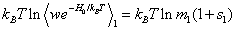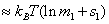(34)

Here, s1 is a normalized deviation from m1. Then, the variance of wexp(-H0/kBT) is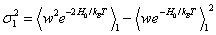(35)

From the above, when taking n1 samples of the λ = 1 ensemble, the variance of Eq. (34) is (kBT)2σ12/m12n1  according to the central limit theorem. The same discussion is valid for the second term of the last line in Eq. (33). Hence, the assessment function for determining the w is as follows: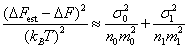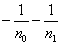(36)

The optimal function w is obtained by minimizing the numerator of the first term, while the denominator is kept constant. With introducing the Lagrange undetermined multiplier Λ corresponding to this constraint, the variation of w leads to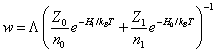(37)

Then, Eq. (33) can be rewritten as: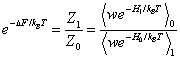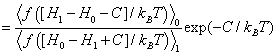(38)

Here,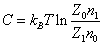(39)

and the function f is the Fermi distribution function: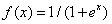(40)

By eliminating Z1/Z0 with Eq. (38) and Eq. (39), the following equation is obtained: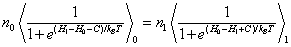(41)

C is obtained by determining the intersection of the two curves corresponding to the left-side function and right-side function with respect to C. The free energy change can be directly determined from Eq. (39):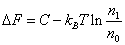(42)

Shirts et al.  re-formulated the BAR method as a maximum likelihood estimation (MLE), which clarifies the spirit of this method. The starting point of their derivation is the Crooks equation ,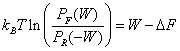(43)

It should be noted that the Crooks equation assumes that the time evolution of the system is discrete and the dynamics are Markovian. In Eq. (43), PF(W) and PR(W) mean the probabilities that the work W is done in the forward (F) and reverse (R) transitions, respectively. Here, PF(W) = P(W|F) can be regarded as a conditional probability that the work W is done when the non-equilibrium transition is forward. Similarly, PR(-W) = P(W|R) can be regarded as a conditional probability that the work -W is done when the non-equilibrium transition is reverse. Note that in the conditional probability form the sign of work is defined in the forward transition.

Because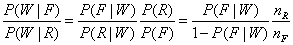is obtained, Eq. (43) can be rewritten as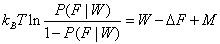(44)

Here, M=kBTln(nF/nR), where nF and nR are the numbers of samples of forward transitions and reverse transition, respectively. Therefore, when one work W is obtained, the probabilities of receiving it from forward transition and reverse transition are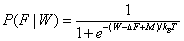(45)

and(46)

respectively. Therefore, the probability that given works of Wi (i=1.. nF) are obtained from forward transitions and the works of Wj (j=1.. nR) from reverse transition is written as: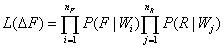(47)

with using ΔF as a parameter. The most likely value of ΔF is obtained by maximizing the likelihood function, Eq. (47). Here, lnL is maximized, but not L. Then, from the stationary condition,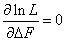(48)

one obtain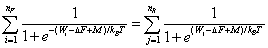(49)

When the work W is done in the instantaneous adiabatic transition, W = ΔV. Then, Eq. (49) becomes identical to Eq. (41). In the BAR method, ΔF is given as the most likely value.

In the case of an extreme condition of nF>>nR, Eq. (49) can be rewritten as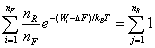(50)

Thus, one obtain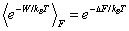(51)

The basic formula of the BAR method encompasses the exponential function average (Jarzynski equality), Eq. (51). In the instantaneous adiabatic process, Eq. (51) becomes equivalent to the FEP method [Eq. (11)], and the original Bennett’s formula [Eq. (41)] encompasses the FEP method.

At the beginning of this subsection, we intuitively mentioned that the advantage of the BAR method is to utilize the works both for forward and reverse transitions. In fact, the ratio nF/nR greatly affects the accuracy. As shown in Fig. 2 of Bennett’s original paper , the free energy calculation becomes most stable and precise, where nF/nR ≈1. However, the computational cost for the calculation of the transitions in both the two directions is not much higher than the cost of calculating one-way transition. This is because in performing the MD simulations for λ = λi, not only the work for the transition to the state of λ = λi+1 (W = ΔV), but also the work for the transition to the state of λ = λi-1 can be calculated simultaneously. Thus, both from the computational and theoretical viewpoints, we can consider that the BAR method is more advantageous than the exponential function averaging method.

2.6. Discussion

In this paper, we have discussed the TI method, the FEP method and the BAR method as important theoretical foundations of the free energy calculation. Interestingly, these methods can be interpreted as the relation between the free energy difference and works associated with state transitions. For example, whereas Zwanzig’s derivation of the FEP method is simply mathematical, the Jarzynski equality can lead to the FEP equation as an exponential function average of work done in instantaneous adiabatic transition. On the other hand, for the quasi-static process, the Jarzynski equality provides the TI method. Also, the Crooks equation leads to the BAR method, which claims that the free energy difference can be obtained via the MLE evaluation of the set of works in forward and reverse transitions. In the case of sampling forward transitions extremely more, the BAR method becomes equivalent to the exponential function average.

As described above, it is very important to consider what physical scenario is hypothesized in the calculation method. If the physical scenario is not reproduced in the MD simulation, the accuracy of the free energy difference prediction should be low. Therefore, in estimating the binding free energy in an actual drug design process, it is important to carefully check whether the physical scenario is reproduced or not.

In fact, it is often observed that an MD simulation is not carried out in the expected way. For example, the problem of Hamiltonian lag frequently experienced in the slow growth method is an issue due to MD simulation breaking the quasi-static process assumed in the slow growth method. When Hamiltonian lag occurs, the prediction of free energy change will not be reliable.

Similar phenomenon appears also in the calculation of the free energy landscape (PMF, potential of mean force) . We investigated the free energy profile of an antigen-antibody system along the dissociation pathway obtained via steered MD (SMD) method and found that the dissociation free energy change is largely dependent on the dissociation route. The fact that the dissociation pathway had a higher free energy change than another dissociation pathway indicates that the former pathway lead the system to a metastable state instead of an equilibrium dissociation state, meaning that the internal structure of the proteins should become distorted. Although it was assumed that the SMD method naturally dissociate the antigen-antibody complex, the dissociation pathway is deviated from a natural dissociation pathway in the actual SMD calculations because the separation speed is artificially fast.

To avoid this problem, the multi-step targeted MD (mTMD) method has been developed to determine a dissociation pathway with fixing the internal structure. Along the dissociation pathway derived with the mTMD method, the free energy change was significantly lower than that along the SMD dissociation pathway . Moreover, the free energy difference among the pathways obtained by the mTMD method was much smaller, indicating that the free energy calculations became stable. For the hen egg white lysozyme (HEL)-HyHEL-10 system, the free energy change calculated with the mTMD method was consistent with the experimental results, which indicated the N32LD mutation of the antibody (HyHEL-10) was successfully reproduced. (Fig. 1) Evaluation of the antigen-antibody interaction is still one of the difficult problems, and therefore the methodological development and improvement continue to be active.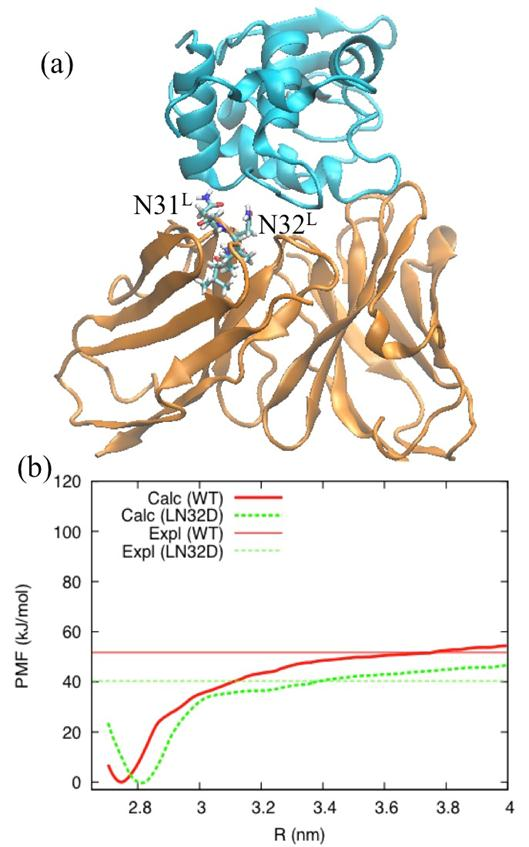Figure 1. (a) Structure of HEL-HyHEL-10 complex. Cyan and orange ribbons represent HEL and HyHEL-10, respectively. (b) PMFs with respect to distance between the centers of mass of HEL and HyHEL-10. Experimental values are the binding free energies, which are closely related to the PMFs. See Ref.  for details.

Recently, we tried to apply an accurate MD-based free energy calculation method, called MP-CAFEE method , to the actual drug development process. The MP-CAFEE method employs most appropriate methods and parameters from various viewpoints (e.g., the BAR method with appropriate λ points). In fact, the calculation accuracy reported so far was very high. For example, for MUP-I (Fig. 2), the calculated binding free energies were -9.0 and -8.2 kcal/mol with IBMP and IPMP, respectively, which were in good agreement with the experimental values (-9.2 and -8.1 kcal/mol, respectively) . Furthermore, the decomposition analysis showed that this difference is mainly due to the van der Waals (vdW) interactions of MUP-I and the ligand. (ΔGvdW = -9.1 and -8.3 kcal/mol for IBMP and IPMP, respectively.)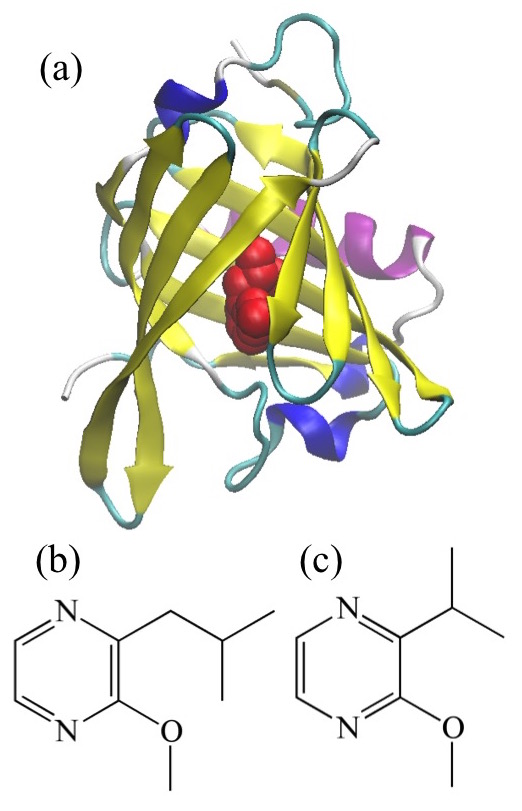Figure 2. (a) Structure of MUP-I. Ribbon is MUP-I and red balls are 3-Isobutyl-2-methoxy pyrazine (IBMP). (b) IBMP. (c) 2-isopropyl-3-methoxy pyrazine (IPMP).

In the MP-CAFEE method, to calculate the binding free energy for a single compound, 768 MD trajectories are required. Because in the drug design it is necessary to calculate binding free energies of a number of compounds, the application of MP-CAFEE method requires huge computational resources. In fact, by utilizing the K computer of RIKEN with the world's first 10 PFlops operation performance, the application has become possible [19, 20].

While the free energy calculation theories are important for the reliable prediction, as discussed above, the accuracy of the force field [19, 21, 22] is also of particular importance. Whatever free energy calculation method we utilize, sampling must be conducted in the correct equilibrium state. However, the long MD simulations with an inaccurate force field, could possibly lead the system to an odd structure. In addition, it may be possible that the quantum effects become important. It was found that the quantum effect cannot be neglected even for heavy atoms [23-25]. Thus, these should be carefully investigated in the future.

3. Molecular Dynamics Simulations of Protein and RNA

In this paper, we discuss the dynamics of biomolecules that can be observed through the latest MD simulation technology. The improvement of MD calculation technology plays an important role not only in the free energy calculations but also in the "high-resolution microscopy", where the observed dynamics should be essential to the understanding of the molecular recognition. In this section, we focus on the achievements of the MD simulation as a "high-resolution microscope". Due to the recent evolution of computer, it becomes easy to investigate dynamics of a small protein with MD simulation. However, there remain many problems in the study of protein complexes and non-protein biomolecules. In the following, after discussion about the predictability of the dynamics and structure distribution in several short peptide systems, we will discuss the structural changes of the antigen-antibody complex as an example of the analysis of protein complexes. Because new functions of RNA were recently discovered , the dynamics of RNA or RNA-protein complex systems becomes an important issue. In the last of this section, we will investigate short RNAs with MD simulation to see the problem in the RNA dynamics.

3.1. Short Peptides

For short peptides, it becomes possible to reproduce the native structure (or conformational distribution) accurately with the current MD calculation technique. For example, Shaw et al.  observed that proteins consisting of up to 80 amino acid residues folded correctly in long MD simulations starting from some unfolded states. For chignolin, consisting of 10 amino acids, we also succeeded to reproduce the folding in a MD simulation (Fig. 3). These results indicate that the folding phenomenon can be simulated with the current all-atom level model, if chaperone, which assists protein folding, is not involved in the real folding.Figure 3. Folding of chignolin. Distance between Cα atoms of the terminal Gly residues (G1 and G10) is shown as a function of time.

The Ala dipeptide (Fig. 4) is not folded into a specific conformation, but is often utilized to examine the model and calculation method as a minimum unit of peptide. In particular, since the conformational distribution was measured by the spectroscopic techniques , it can also be used to verify the accuracy of the force field. The conformational distribution obtained in the MD simulation significantly depends on the employed force field . While for many force fields the distribution of α-type conformation is overestimated, it agrees well with experimental results (Table 1) for the FUJI force field , of which the main-chain dihedral parameters are improved based on the high-level electronic state calculation. This means that even with the same function form, the update of the parameters can improve the force field. (Note that the FUJI force field was employed in all of the MD simulations below, unless otherwise stated.)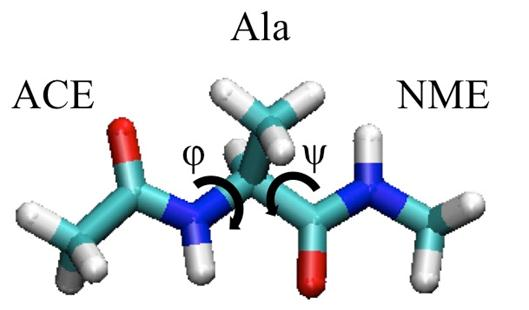Figure 4. Ala dipeptide.

Table 1. Conformational distribution of Ala dipeptide (in percentage).

 αR β PII Others FUJIa 5.9 ± 0.2 22.6 ± 0.2 70.6 ± 0.5 0.8 ± 0.4 ff94a 86.7 ± 1.3 3.0 ± 0.5 9.5 ± 1.0 0.7 ± 0.3 ff99a 88.0 ± 0.6 6.2 ± 0.4 1.9 ± 0.0 3.8 ± 0.3 ff99SBa 26.4 ± 0.5 27.7 ± 0.6 44.0 ± 0.3 1.8 ± 1.0 ff03a 42.0 ± 2.0 19.6 ± 1.0 37.3 ± 1.2 1.1 ± 0.1 IRb 11 29 60 --- Ramanb 9 29 62 ---

a The values were taken from Ref. . bThe experimental values were taken from Ref. .

The influence of the side chain may not be negligible even if the peptide is not folded. To see the side chain effect, MD simulation was performed for three capped tetra-peptides (YYY, FFF, and WWW) . Since the residue Y as well as F and W has a 6-membered carbon ring in the side chain, we characterize the peptide structure with the distance between the centers of mass of the 6-membered carbon rings of the first residue and the second residue. For all the three peptides, there are low peaks at ~0.5 nm (Fig. 5). This corresponds to structures formed by direct interaction between the 6-membered rings, but not a predominant peak. At the dominant peak ~0.8 nm for each peptide, the first residue and the second residue do not interact directly. Because the peaks for all the peptides are similar to one another, they should be mainly affected by the dihedral interaction of the main chain. The slight shift of the WWW peak is due to the fact that the 6-membered rings are farther from the main chain than those of FFF and YYY. In this sense, the interaction effects between adjacent side chains do not seem so relevant.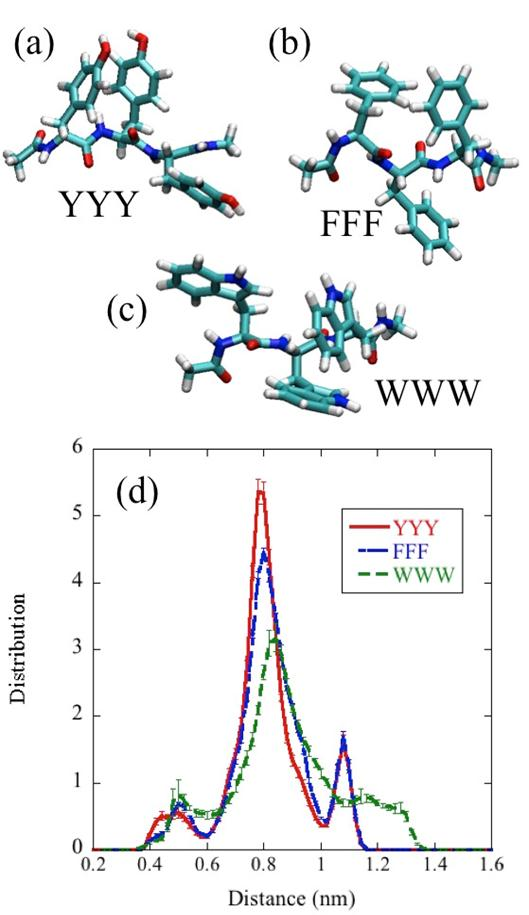Figure 5. (a) YYY. (b) FFF. (c) WWW. (d) Distribution of distance between the first and second six-membered rings. To sample the structural data, five 900 ns MD simulations were conducted for each peptide.

However, when looking into more detail, the right wing (~ 0.9 nm) of the FFF peak is larger than that of YYY. This is due to the effect of OH groups of YYY, which probably involves the third residue. Moreover, the YYY peptide has a small peak around 0.45 nm that cannot be seen in WWW or FFF. This peak should correspond to the formation of the direct hydrogen bond between Y1 and Y2. Thus, we can consider that the structural distribution of the peptide chain is influenced by the sequence of amino acid residues, even if a secondary structure is not formed. Such a point of view will be important to understand the function of the intrinsically disordered protein  at the atomic level.

3.2. Antigen-Antibody Complexes

Although the MD simulation of a simple single protein has become relatively easy, that of a system consisting of several proteins is still very challenging. The difficulty of the protein complex simulation arises not only in the fact that longer time scale is required, but also in the absence of skillful techniques to characterize a protein-protein interface.

One example of the protein complexes is an antibody bound to a protein antigen, where the structure of antigen-antibody interface is considered to be important for the antigen-recognition of the antibody. The antigen-recognition of the antibody not only plays an important role in the immune system, but also becomes a technology for treating intractable diseases such as cancer . Therefore, the understanding of the interfacial effect exerts a spreading effect on a wide range of fields.

B2212A is an antibody that recognizes the Fn3 domain of ROBO1, which is specifically expressed in human hepatocellular carcinoma and is a potential therapeutic target . The X-ray crystallography revealed considerable conformational changes of Y50L of B2212A on binding the Fn3 domain (Fig. 6). As a result of structural change, the hydroxyl group of Y50L forms a hydrogen bond to the main chain oxygen of F68 of Fn3, whereby the antigen-antibody interaction is enhanced. In fact, the MD simulation and thermodynamic analysis confirmed that Y50LA mutation significantly decreased the antigen-antibody interaction energy (or the binding enthalpy). It was found by the MD simulation that this mutation not only leads to a direct effect of losing hydrogen bonding, but also causes non-negligible indirect impact on the binding free energy through subtle adjustment of the interface.Figure 6. ROBO1 Fn3 domain-B2212A Fv complex. Light-colored sticks represent the Y50L conformation in the ROBO1-binding state, while the blue sticks represent the conformation in the free state.

In contrast, no apparent structural change of the HEL-antibody, HyHEL-10, is observed when it binds to HEL (Fig. 1a). However, there is a possibility that the antibody surface in contact with the antigen should have different structure and fluctuation from that in contact with bulk water. To investigate systematically the differences in the structure and fluctuation, we proposed to use the directional analysis of dihedral angles of the main chain . Although the fluctuation in the Cartesian coordinates can be investigated with the root mean square fluctuation (RMSF) method, it is difficult to determine the meaningful average structure and to specify the internal coordinates that affect the RMSF. The directional analysis of the main chain dihedral angle, which overcomes the periodicity problem, enables to calculate the average and fluctuation of dihedral angle systematically. In the directional analysis, the average and variance of the angle property are defined as:(52)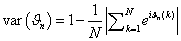(53)

Here, ϑn is a Ramachandran angle (φ or ψ) of the nth residue and k is the index of the sample and we analyzed the 150–210 ns time region of six MD trajectories for each state. Δ(property) = (property of HEL-bound antibody) − (property of free antibody) is calculated to evaluate the difference. In this study, we focus on a part of light chain (from S28L to Y36L). (See Table 2.) In the RMSF analysis, the fluctuation of N32L, N31L and G30L is diminished by the direct contact with its antigen, while the fluctuation of N31L and G30L is significantly decreased also in directional analysis of the ψ and φ angles. However, the correlation between the RMSF value and the variance of the dihedral angle is not high, and the correlation coefficients (R2) are 0.3 and 0.1 for φ and ψ, respectively. For example, the changes of variances for φ and ψ of N32L are not as large as those of N31L, whereas the fluctuation is greatly decreased with respect to ψ of H34L and φ of W35L. Interestingly, we found that the fluctuation of φ of H34L is significantly enhanced by the antigen-binding.

Table 2. Difference of structural and dynamic properties a.

 Residue No. ΔRMSF (×10-2Å) ΔφAVE (deg) ΔψAVE (deg) Δvar(φ) (×102) Δvar(ψ) (×102) S28L −0.4 ± 0.7 −0.5 ± 0.4 +1.5 ± 0.6 −0.02 ± 0.01 −0.10 ± 0.09 I29L −3.5 ± 0.6 −2.8 ± 0.5 −2.1 ± 0.5 −0.11 ± 0.09 −0.34 ± 0.05 G30L −4.1 ± 0.4 +2.6 ± 0.5 +6.0 ± 0.8 −0.34 ± 0.02 −0.51 ± 0.04 N31L −4.4 ± 0.8 −6.0 ± 0.5 +3.1 ± 0.4 −1.65 ± 0.07 −0.77 ± 0.02 N32L −4.8 ± 0.6 +2.9 ± 0.2 −2.1 ± 0.9 −0.22 ± 0.01 +0.17 ± 0.09 L33L −2.2 ± 0.3 −1.6 ± 0.7 −5.5 ± 0.8 +0.17 ± 0.09 +0.15 ± 0.06 H34L −0.3 ± 0.5 +7.7 ± 1.4 +4.3 ± 1.0 +0.54 ± 0.11 −0.53 ± 0.15 W35L −0.7 ± 0.7 −3.3 ± 1.9 −1.2 ± 1.3 −0.61 ± 0.20 +0.15 ± 0.08 Y36L +0.1 ± 0.9 +2.4 ± 1.2 +0.7 ± 1.1 +0.22 ± 0.10 −0.11 ± 0.12

a The values were taken from Ref. 

The directional analysis allows us to determine the average angle (Table 2). Significant changes are clearly observed in φ of H34L, ψ of G30L, φ of N31L and ψ of L33L on average. Note that the binding effects on the mean dihedral angles appear randomly. Even though an apparent structural change does not occur as in the case of B2212A, we can detect and discuss the change in the mean structure and fluctuation by carefully analyzing the MD simulation.

3.3. Short RNAs

Recently, a novel function of non-coding RNA (ncRNA), which is not translated into protein, has been discovered . A certain ncRNA recognize and binds to a protein, then influencing transcription. For example, Kurokawa et al.  found the pncRNA-D, an ncRNA binding to TLS, is deeply involved in the control of gene expression. Thus, the study on the structure and dynamics of the RNA-protein complex is important for understanding the RNA-protein interaction. Importantly, structure and dynamics of RNA are significantly different from those of a peptide. Here, in order to clarify the features of RNA, MD simulations of four short RNAs (AAA, GGG, CCC, and UUU) are discussed . (Note that the bsc0 force field  was used here.)

Since all the nucleic acid bases (A, G, C, and U) include aromatic 6-membered rings, the RNA structure is characterized by distance between centers of mass of the 6-membered rings of the first base and second base (Fig. 7). While in the cases of the peptides (Fig. 5), structures where the side chains directly interact to each other are not often observed, the base stacks are effectively formed and the direct interaction of adjacent bases is predominant in the cases of short RNAs. In fact, the main peak of the distribution of distance (Fig. 7) can be observed at 0.45-0.5 nm. Furthermore, although the sampling of RNA structures is performed for the same time period of MD simulation as in the case of peptides, the standard errors of the distributions for the RNAs are larger than those for the peptides. The reason is that formation and breaking of the interaction between the adjacent bases are slow, which is one of the factors that render more difficult the MD simulations of RNA. Even for the short RNAs discussed here, we have already performed five MD simulations for 900 ns (i.e., totally 4.5 μs) to suppress the errors to this extent.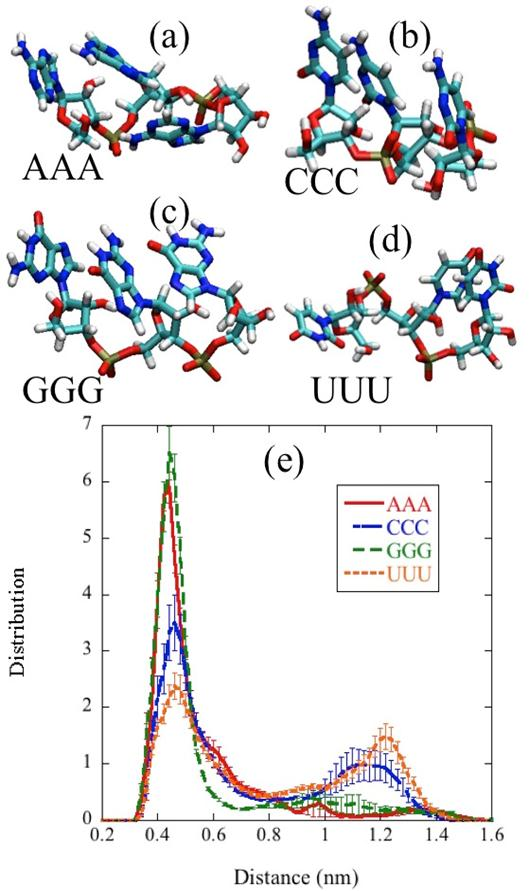Figure 7. (a) AAA. (b) CCC. (c) GGG. (d) UUU. (e) Distribution of distance between the first and second six-membered rings. To sample the structural data, five 900 ns MD simulations were conducted for each peptide.

The structure and dynamics characterized by the distance between the 6-membered rings of two adjacent bases depend largely on the base type. In the cases of purines (A and G), the faces of the base rings prone to form the stack structure more strongly. In the cases of pyrimidines (C and U), the stack face of the base is small and the stack structure is less stabilized. Therefore, the positions of main peak of the distance distributions for CCC and UUU are slightly shifted to right. In addition, the stack structure is more frequently collapsed (distance > 0.8nm) in CCC and UUU than in AAA and GGG. In more detail, although both of A and G are purines, the distribution in AAA is different from that in GGG. Although C and U are pyrimidines, the structural distributions of UUU and CCC are different to each other. This means that the difference of modification of base also affects the structure and fluctuation in short RNAs. For the long RNA, many informatics-based prediction methods have been proposed, some of which focus on the secondary structure with assigning Watson-Crick base pairs in the RNA chain . In contrast, the short RNAs studied here do not form a Watson-Crick base pair, and therefore the dynamics under the influence of the interaction between adjacent bases is predominant. In addition, we consider that the study of the RNA dynamics is crucial for the understanding of the molecular function of long RNA and RNA-protein complexes, because the structural change of RNA reflects the individual nature of the nucleic acid base sensitively. Therefore, the RNA research by MD simulation will become increasingly important in the future.

4. Concluding Remarks

In this review, we discussed how molecular recognition, the foundation of biological activities, could be approached with MD simulation. This is still a very challenging subject and therefore many ideas have been made from theoretical and practical viewpoints. For the theoretical aspects, we described the development of the free energy calculation theories and discussed the recent developments. The theory of free energy calculation continues to evolve under the influence of the latest statistical mechanics. For example, by using the Jarzynski equality, physical processes can be corresponded to the free energy calculation to suggest suitable conditions in the FEP and TI calculations. From the practical aspects, we discussed how the conformational dynamics of the biomolecule could be analyzed with long-time MD simulations, which become available due to the technological evolution of computer. Although several simple examples were considered here, the structural change dynamics are often essential to the molecular recognition. In flexible protein systems with more complex energy landscape, further development of effective methods is required to realize the highly accurate prediction of free energy. It may not be sufficient to simply enable a long time simulation, but the effective use of efficient techniques, such as generalized ensemble method , umbrella sampling method  and Markov state model method , are also important.

The "fragility" of the protein structure should be paid due attention when using the MD simulation. For example, the motion of a ligand bound to a protein is characterized in the protein frame axes usually defined by the entire protein. However, when some domain of the protein is greatly unfolded in the MD simulation, the protein frame moves and consequently affects apparent movement of the ligand due to the large motion of the unfolded domain. In order to avoid such an illusion, it is necessary to define the coordinate axes only with the skeletal core (the protein domain with small fluctuation) .

MD simulation is a general-purpose technique, capable of investigating not only biomolecules but also macromolecular materials. In this review, although we addressed as many topics as possible, still many subjects were left uncovered. For these subjects, see Refs. [42,43]. For biomolecules, it will become a challenging research to analyze functions of protein complexes and nucleic acid-protein complexes. Although not covered in this paper, the study of lipid bilayer will also become important. In fact, the lipid bilayer [44,45] as well as proteins embedded in a membrane  also plays an important role e.g., in the proton transport.

Finally, we emphasized that the success of MD calculations greatly depends on the accuracy of the force field. Even if sufficiently long MD simulations were performed, reliable prediction might not be provided with an inaccurate force field. The force field development is a sober work, but not necessarily easy. While we need to pay attention to the consistency of the model over the whole system, we have to model individual molecules, which have often very different characteristics from one another. For example, since the peptide main chain forms an intramolecular hydrogen bond, it is necessary to carefully select an appropriate electronic state calculation method for deriving the dihedral angle parameters. If the potential energy is overestimated only by 1 kcal/mol, the existence probability becomes about five times smaller decrease, which indicates the quantitative modeling is necessary. Thus, it will be important to further improve the biomolecules force field in the future.

Many methods effectively utilizing MD simulations continue to be developed, inspired by various applications and associated with the advancement of the relevant theories and techniques. We expect that such developments will expand frontier of research on the molecular recognition.

Acknowledgements

This study was mainly supported by grant-in-aids for Scientific Research (JSPS KAKENHI (S)15H05752 and (C)15KT0103) and the SIP project [Structural Materials for Innovation (SM4I)]. This research used computational resources of K (AICS) and TSUBAME2.5 (Tokyo Tech) through the HPCI System Research Project (hp140200, hp150056, and hp160077). The author also thanks the HPCI strategic program field 1 (hp140228, and hp150230), Innovative Drug Discovery Infrastructure through Functional Control of Biomolecular Systems [Priority Issue 1 in Post-K Supercomputer Development (hp160223)], and AMED project (15ck0106029h0002). The author also wishes to acknowledge fruitful discussion with Prof. R. Kurokawa, Prof. T. Kodama, Prof. T. Hamakubo, Prof. H. Fujitani, and Dr. Y. Takamatsu.

References

1. Andreani J and Guerois R: Evolution of protein interactions: from interactomes to interfaces. Arch Biochem Biophys 2014, 554:65-75.
2. Jankowsky E and Harris ME: Specificity and nonspecificity in RNA-protein interactions. Nat Rev Mol Cell Biol 2015, 16:533-544.
3. Mortier J, et al.: The impact of molecular dynamics on drug design: applications for the characterization of ligand-macromolecule complexes. Drug Discov Today 2015, 20:686-702.
4. Shaw DE, et al.: Atomic-level characterization of the structural dynamics of proteins. Science 2010, 330:341-346.
5. Shuman CF, Hamalainen MD, and Danielson UH: Kinetic and thermodynamic characterization of HIV-1 protease inhibitors. J Mol Recognit 2004, 17:106-119.
6. Mobley DL, Chodera JD, and Dill KA: On the use of orientational restraints and symmetry corrections in alchemical free energy calculations. J Chem Phys 2006, 125:084902.
7. Chodera JD, et al.: Alchemical free energy methods for drug discovery: progress and challenges. Curr Opin Struct Biol 2011, 21:150-160.
8. Jorgensen WL, et al.: Efficient Computation of Absolute Free-Energies of Binding by Computer-Simulations - Application to the Methane Dimer in Water. J Chem Phys 1988, 89:3742-3746.
9. Frenkel D and Smit B: Understanding Molecular Simulation (2nd Ed.). 2002: Academic Press, San Diego.
10. Pearlman DA and Kollman PA: The Lag between the Hamiltonian and the System Configuration in Free-Energy Perturbation Calculations. J Chem Phys 1989, 91:7831-7839.
11. Zwanzig RW: High-Temperature Equation of State by a Perturbation Method.1. Nonpolar Gases. J Chem Phys 1954, 22:1420-1426.
12. Jarzynski C: Nonequilibrium equality for free energy differences. Phys Rev Lett 1997, 78:2690-2693.
13. Jarzynski C: Nonequilibrium work theorem for a system strongly coupled to a thermal environment. J Stat Mech-Theory E 2004:P09005.
14. Bennett CH: Efficient Estimation of Free-Energy Differences from Monte-Carlo Data. J Comput Phys 1976, 22:245-268.
15. Shirts MR, et al.: Equilibrium free energies from nonequilibrium measurements using maximum-likelihood methods. Phys Rev Lett 2003, 91:140601.
16. Crooks GE: Path-ensemble averages in systems driven far from equilibrium. Phys Rev E 2000, 61:2361-2366.
17. Yamashita T and Fujitani H: On accurate calculation of the potential of mean force between antigen. and antibody: A case of the HyHEL-10-hen egg white lysozyme system. Chem Phys Lett 2014, 609:50-53.
18. Fujitani H, et al.: Direct calculation of the binding free energies of FKBP ligands. J Chem Phys 2005, 123:084108.
19. Yamashita T, et al.: The Feasibility of an Efficient Drug Design Method with High-Performance Computers. Chem Pharm Bull 2015, 63:147-155.
20. Yamashita T, et al.: Molecular Dynamics Simulation-Based Evaluation of the Binding Free Energies of Computationally Designed Drug Candidates: Importance of the Dynamical Effects. Chem Pharm Bull 2014, 62:661-667.
21. Fujitani H, et al.: High performance computing for drug development on K computer. J Phys Conf Ser 2013, 454:012018.
22. Yamashita T: Improvement in Empirical Potential Functions for Increasing the Utility of Molecular Dynamics Simulations. JPS Conf Proc 2015, 5:010003.
23. Yamashita T and Kato S: Regularity in highly excited vibrational dynamics of NOCl (X(1)A(')): Quantum mechanical calculations on a new potential energy surface. J Chem Phys 2003, 119:4251-4261.
24. Yamashita T and Kato S: Excited state electronic structures and dynamics of NOCl: A new potential function set, absorption spectrum, and photodissociation mechanism. J Chem Phys 2004, 121:2105-2116.
25. Yamashita T and Kato S: Resonance Raman spectra of NOCl: Quantum dynamics study. Chem Phys Lett 2005, 405:142-147.
26. Lindorff-Larsen K, et al.: How Fast-Folding Proteins Fold. Science 2011, 334:517-520.
27. Grdadolnik J, et al.: Populations of the three major backbone conformations in 19 amino acid dipeptides. Proc Natl Acad Sci USA 2011, 108:1794-1798.
28. Fujitani H, et al.: High-Level ab Initio Calculations To Improve Protein Backbone Dihedral Parameters. J Chem Theory Comput 2009, 5:1155-1165.
29. Yamashita T: Effects of side chain on short biopolymer conformation. unpublished.
30. Oldfield CJ and Dunker AK: Intrinsically Disordered Proteins and Intrinsically Disordered Protein Regions. Annu Rev Biochem 2014, 83:553-584.
31. Steiner M and Neri D: Antibody-Radionuclide Conjugates for Cancer Therapy: Historical Considerations and New Trends. Clin Cancer Res 2011, 17:6406-6416.
32. Nakayama T, et al.: Structural features of interfacial tyrosine residue in ROBO1 fibronectin domain-antibody complex: Crystallographic, thermodynamic, and molecular dynamic analyses. Protein Sci 2015, 24:328-340.
33. Yamashita T: On the Accurate Molecular Dynamics Analysis of Biological Molecules. AIP Conf. Proc. (in press).
34. Hu WQ, Alvarez-Dominguez JR, and Lodish HF: Regulation of mammalian cell differentiation by long non-coding RNAs. Embo Rep 2012, 13:971-983.
35. Wang X, et al.: Induced ncRNAs allosterically modify RNA-binding proteins in cis to inhibit transcription. Nature 2008, 454:126-130.
36. Perez A, et al.: Refinement of the AMBER force field for nucleic acids: improving the description of alpha/gamma conformers. Biophys J 2007, 92:3817-3829.
37. Hamada M and Asai K: A Classification of Bioinformatics Algorithms from the Viewpoint of Maximizing Expected Accuracy (MEA). J Comput Biol 2012, 19:532-549.
38. Okamoto Y: Generalized-ensemble algorithms: enhanced sampling techniques for Monte Carlo and molecular dynamics simulations. J Mol Graph Model 2004, 22:425-439.
39. Kastner J: Umbrella sampling. Comput Mol Sci 2011, 1:932-942.
40. Lane TJ, et al.: Markov State Model Reveals Folding and Functional Dynamics in Ultra-Long MD Trajectories. J Am Chem Soc 2011, 133:18413-18419.
41. Sakano T, et al.: Molecular dynamics analysis to evaluate docking pose prediction. Biophys Physicobiol 2016, 13:181–194.
42. Dror RO, et al.: Biomolecular Simulation: A Computational Microscope for Molecular Biology. Annu Rev Biophys 2012, 41:429-452.
43. Perilla JR, et al.: Molecular dynamics simulations of large macromolecular complexes. Curr Opin Struct Biol 2015, 31:64-74.
44. Yamashita T and Voth GA: Properties of Hydrated Excess Protons near Phospholipid Bilayers. J Phys Chem B 2010, 114:592-603.
45. Yamashita T: Properties of a Hydrated Excess Proton Near the Cholesterol-Containing Phospholipid Bilayer. JPS Conf Proc 2014, 1:013086.
46. Yamashita T and Voth GA: Insights into the Mechanism of Proton Transport in Cytochrome c Oxidase. J Am Chem Soc 2012, 134:1147-1152.

 Contents 1. 2. 2.1. 2.2. 2.3. 2.4. 2.5. 2.6. 3. 3.1. 3.2. 3.3. 4.
Article ToolsAbstractPDF(1372K)